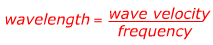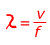0
102

Wavelength Formula

Wavelength is the distance between the crests of a wave. Many different things can move like waves, like strings, water, the air (sound waves), the ground (earthquakes), and light can be treated as a wave. Wavelength is represented with the Greek letter lambda: λ. It is equal to the velocity of the wave, divided by the frequency. Wavelength is expressed in units of meters (m).Answer: The wave velocity v = 340 m/s, and the frequency f = 20.0 cycles/s. The wavelength ? can be found using the equation:The wavelength of the sound is 17 meters.